#Function Repository Resource:

# DropSmallestBy

Drop the smallest values from a list based on a function

Contributed by: Sander Huisman
 ResourceFunction["DropSmallestBy"][list,f,n] drops the n elements ei from list for which f[ei] is smallest. ResourceFunction["DropSmallestBy"][f,n] represents an operator form of ResourceFunction["DropSmallestBy"] that can be applied to an expression.

## Examples

### Basic Examples (3)

Drop the smallest two values determined by their magnitude:

 In:=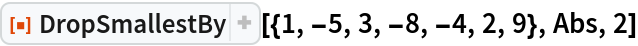Out=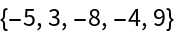Drop the smallest two complex values determined by their magnitude:

 In:=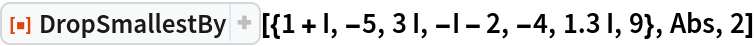Out=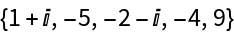Use the operator form, by first creating an operator op:

 In:=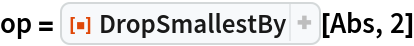Out=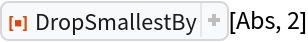Apply the operator:

 In:=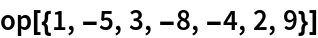Out=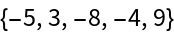### Scope (2)

When n=0, nothing is deleted:

 In:=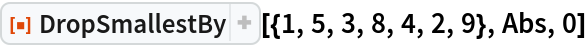Out=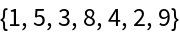A negative number deletes the largest n numbers:

 In:=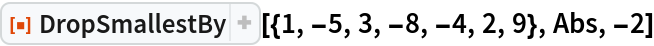Out=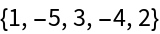### Properties and Relations (2)

If n is larger than the length of the list, an empty list is returned:

 In:=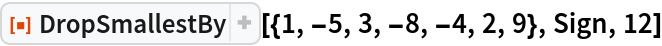Out=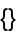DropSmallestBy and TakeSmallestBy are complementary:

 In:=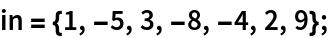In:=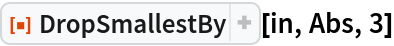Out=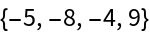In:=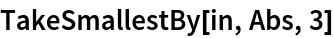Out=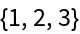SHuisman

## Version History

• 1.0.0 – 02 April 2020